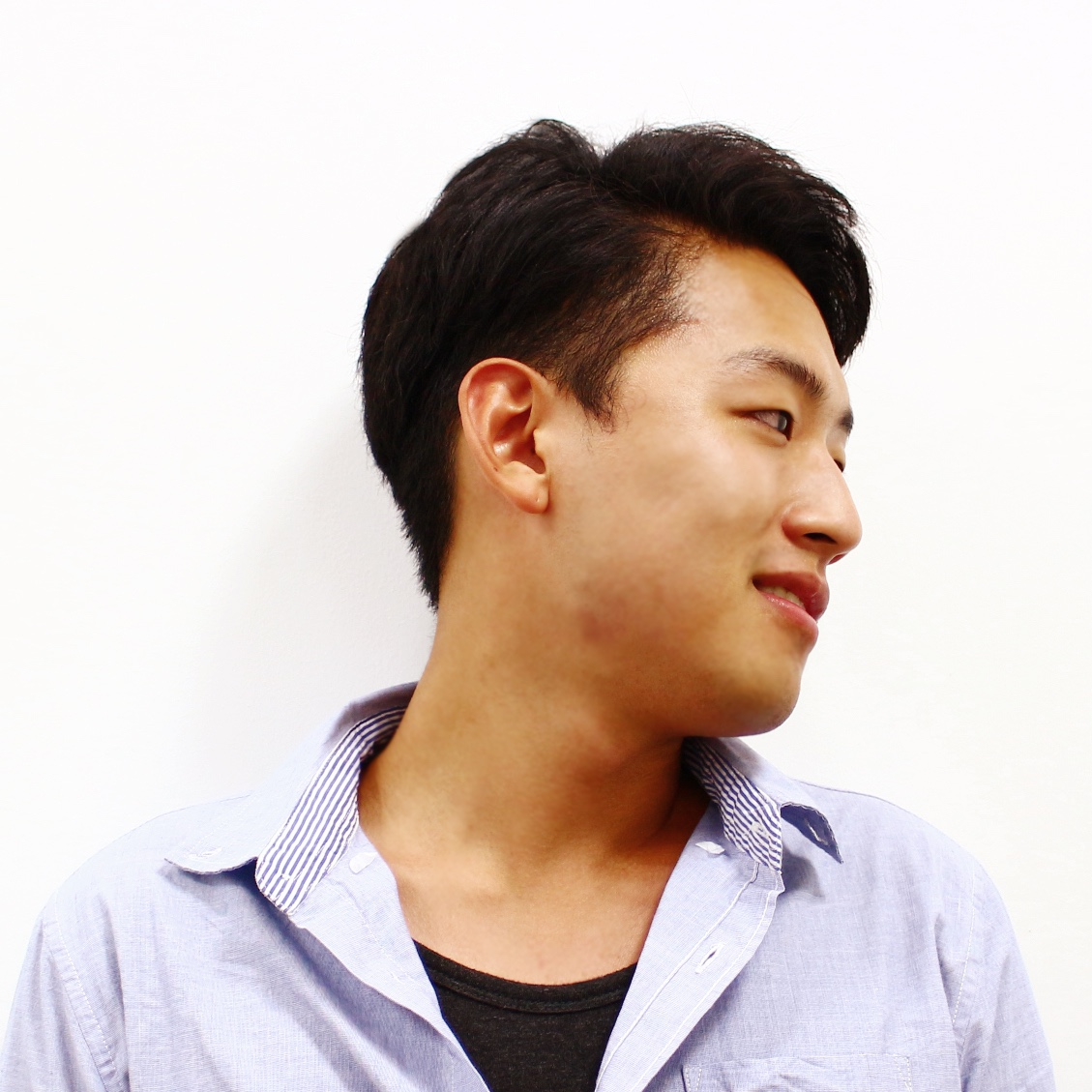/ Deep Learning

# Normalization layer

What is Batch Normalization?
Instance Normalization?
Conditional Batch Normalization?
Conditional Instance Normalization?

Batch Normalization is first introduced by Sergey Ioffe, Christian Szegedy. It increased image classification performance significantly.

• arxivInterested in "Conditional Batch Normalization (CBN)", here's wrap up of normalization layers.

refer to "Arbitrary Style Transfer in Real-time with Adaptive Instance Normalization"
Well compared in this paper.

## Batch Normalization (BN)

$$\text{BN}(x)=\gamma (\frac{x-\mu(x)}{\sigma(x)})+\beta$$

$\gamma, \beta \in \mathbb{R}^C$ are affine parameters learned from data;
mean ad standard deviation, computed across batch size and spatial dimensions indenpedently for each feature channel

$\mu_c(x)=\frac{1}{NHW}\sum\limits_{n=1}^N \sum\limits_{h=1}^H \sum\limits_{w=1}^W x_{nchw}$
$\sigma_c(x)=\sqrt{\frac{1}{NHW}\sum\limits_{n=1}^N \sum\limits_{h=1}^H \sum\limits_{w=1}^W (x_{nchw}-\mu_c(x))^2+\epsilon}$

## Instance Normalization (IN)

$$\text{IN}(x)=\gamma (\frac{x-\mu(x)}{\sigma(x)})+\beta$$

$\mu_{nc}(x)=\frac{1}{HW} \sum\limits_{h=1}^H \sum\limits_{w=1}^W x_{nchw}$
$\sigma_{nc}(x)=\sqrt{\frac{1}{HW} \sum\limits_{h=1}^H \sum\limits_{w=1}^W (x_{nchw}-\mu_{nc}(x))^2+\epsilon}$

While BN takes average among channels, IN takes average in each channels. Thus, each channels won't be affected. Image generation is very dependent on channels compared to image classification. IN takes very important parts in image generation. State-of-the-art such as CycleGAN, StarGAN ... uses IN instead of BN.

In my opinion, BN is good for discrminative job and IN for generative job.

Here's difference between BN and IN## Conditional Batch Normalization (CBN)

First instoduced from Modulating early visual processing by language.
in NIPS2017. CBN is introduced from Auron Courville's lab.Also predict delta value of $\mu$ and $\sigma$ on BN. Thus, $\mu$ and $\sigma$ of BN will be conditioned to some other Neural Net (questions, query, ...).

## Conditional Instance Normalization (CIN)

$$\text{CIN}(x;s)=\gamma^s (\frac{x-\mu(x)}{\sigma(x)})+\beta^s, s \in {1,2,3,...,S}$$
Surprisingly, the network can generate images in completely different styles by using the same convolutional parameters but different affine parameters in IN layers.

CIN is would be good for conditional image generation (sytle transfer for given style. Compute style and use the $\mu$ and $\sigma$ for image generation.)

Ulyanov et al.  attribute the success of IN to its invariance to the contrast of the content image. However, IN takes place in the feature space, therefore it should have more profound impacts than a simple contrast normalization in the pixel space. Perhaps even more surprising is the fact that the affine parameters in IN can completely change the style of the output image.

AdaIN has no learnable affine parameters. Instead, it adaptively computes the affine parameters from the style input:
$$\text{AdaIN}(x;s)=\sigma(y) (\frac{x-\mu(x)}{\sigma(x)})+\mu(y)$$
in which we simply scale the normalized content input with $\sigma(y)$, and shift it with $\mu(y)$.

In my opinion, we already use the term Conditional Normalization instead of Adative Normalization.

# Applications

## FiLM: Visual Reasoning with a General Conditioning Layer

by Ethan Perez, Florian Strub, Harm de Vries, Vincent Dumoulin, Aaron Courville
AAAI 2018. Code available at this http URL . Extends arXiv:1707.03017.

This work outperforms Deepmind's "relation network" VQA task.

github

## Conditional Instance Normalization used from "A LEARNED REPRESENTATION FOR ARTISTIC STYLE"

Vincent Dumoulin & Jonathon Shlens & Manjunath Kudlur, Google BrainOutputs multiple style trasfered output with a single network.

## Augmented CycleGAN: Learning Many-to-Many Mappings from Unpaired Data

by Amjad Almahairi, Sai Rajeswar, Alessandro Sordoni, Philip Bachman, Aaron Courville

Submitted to ICML2018, arXiv:1802.10151v1

Uses CIN for many-to-many mapping.#### Junho Cho

Integrated Ph.D course and Interested in Computer Vision, Deep Learning. For more information, tmmse.xyz/junhocho/